# VBA Current Region

This tutorial will explain the how to use Current Region in VBA.

The CurrentRegion property in VBA is a way to select all the cells that are contained within a Range that you might want to work with. As long as the cells within the range are all consecutive or ‘touching’ each other, the CurrentRegion will select every cell in the region.

## CurrentRegion Syntax

The syntax is very simply:

``Range("E11").CurrentRegion.Select``

where you would specify the cell whose current region you wished to select and then use the CurrentRegion.Select property to select the Range of Cells.

## Obtain the Current Region

Consider the following worksheet.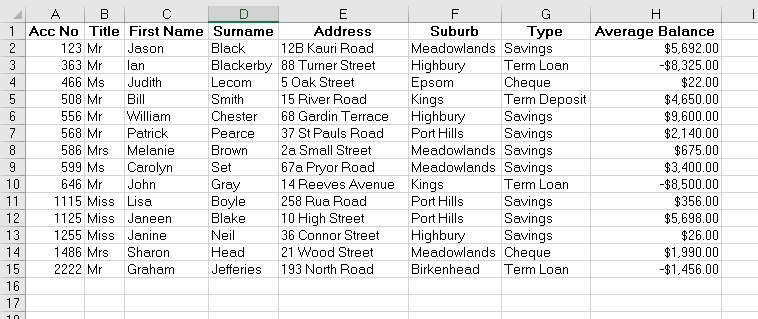The following Sub Procedure would select all the cells within the Excel table.

``````Sub FindCurrentRegion()
Dim rng As Range
'set the range to be Cell E11
Set rng = Range("E11")
'select the current region
rng.CurrentRegion.Select
End Sub``````

If we run the routine, all the cells in the CurrentRegion of cell E11 will be selected.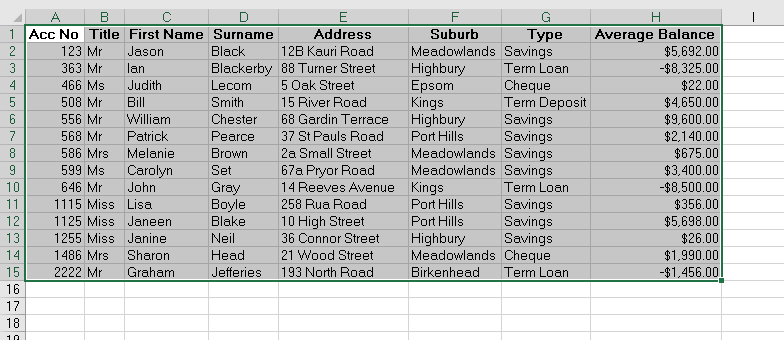If we remove the contents of the adjoining cells, and re-run the routine, the following will be selected as the current region.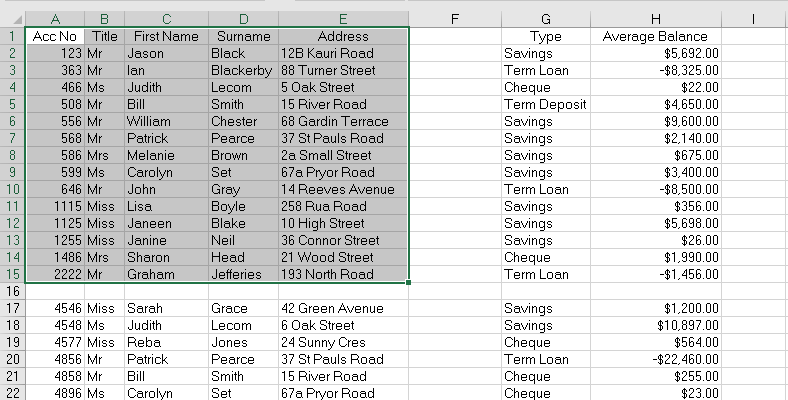However if we remove even more data, we could end up with the example below for the Current Region of Cell E11.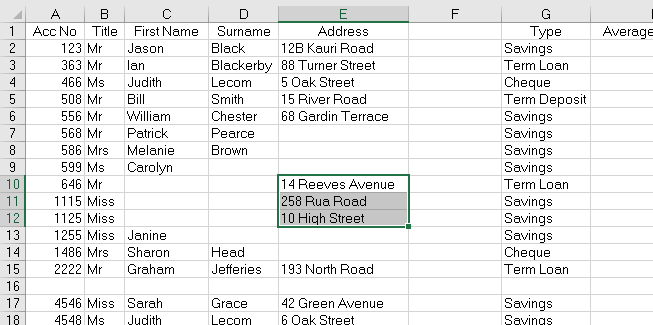Putting information ack into D13, we end up with the following: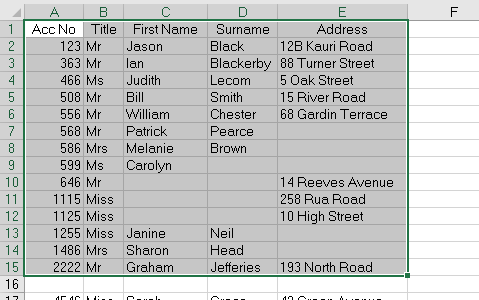The CurrentRegion therefore returns another range object defined by the smallest combination of occupied columns and rows that surround the Range you have supplied.

## Counting the Rows and Columns in the Current Region

We can use CurrentRegion to count the rows and columns.

``````Sub FindCurrentRegion()
Dim rng As Range
Dim iRw As Integer
Dim iCol As Integer
'set the range
Set rng = Range("E11")
'count the rows
iRw = rng.CurrentRegion.Rows.Count
'count the columns
iCol = rng.CurrentRegion.Columns.Count
'show the result in a message box
MsgBox ("We have " & iRw & " rows and " & iCol & " columns in our current region")
End Sub``````

If we run the procedure, the following message box will appear.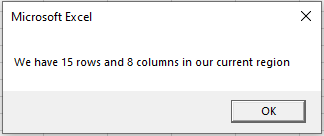## Clearing the Current Region

We can also use the current region property to clear the range of cells.

``````Sub ClearCurrentRegion()
Dim rng As Range
'set the range
Set rng = Range("E11")
rng.CurrentRegion.Clear
End Sub``````

## Assigning the Current Region to a Variable

We can also assign the entire Current Region to a range variable, and then use that range variable to manipulate the cells – be it formatting the cells, sorting the cells etc..

``````Sub AssignCurrentRegionToVariable()
Dim rng As Range
'set the range to be the current region of E11
Set rng = Range("E11").CurrentRegion
'color the background and text
rng.Interior.Pattern = xlSolid
rng.Interior.Color = 65535
rng.Font.Bold = True
rng.Font.Color = -16776961
End Sub``````

If we run the procedure above, we will end up with a worksheet as shown below!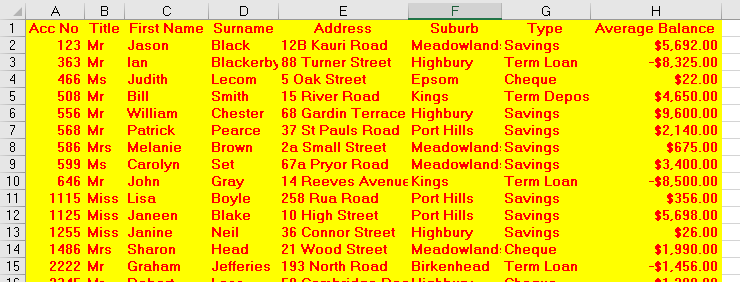## Get the Start and End Cells in the Current Region

With slightly more complicated code, we can get the first cell and last cell in a Current Region.

``````Sub GetStartAndEndCells()
Dim rng As Range
Dim iRw As Integer
Dim iCol As Integer
Dim iColStart, iColEnd, iRwStart, iRwEnd As String
'set the range variable to be the current region of E11
Set rng = Range("E11").CurrentRegion
'set the start column for the range
iColStart = rng.Column
'get the end column for the range
iColEnd = iColStart + (rng.Columns.Count - 1)
'get the start row for the range
iRwStart = rng.Row
'get the end row for the range
iRwEnd = iRwStart + (rng.Rows.Count - 1)
'display the address of the start and end rows and columns in a message box
MsgBox ("The Range start at " & Cells(iRwStart, iColStart).Address & " and ends at " & Cells(iRwEnd, iColEnd).Address)
End Sub``````

When we run the code above, the following message box will appear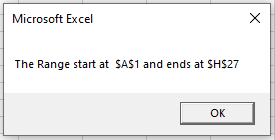Stop searching for VBA code online. Learn more about AutoMacro - A VBA Code Builder that allows beginners to code procedures from scratch with minimal coding knowledge and with many time-saving features for all users!